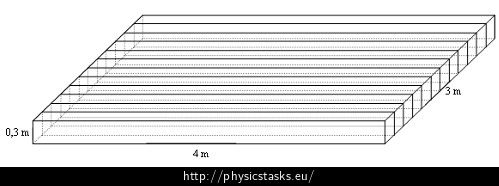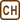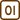## Shipwreck Survivors

### Task number: 1163

Two shipwreck survivors put to sea in the raft which they had made from beams. The length of the raft is 4 m, the width is 3 m, the height is 30 cm (see the figure). The density of the wood, which the raft is made of, is 600 kg/m3.a) Will the raft sink with the shipwreck survivors whose weight altogether with the supplies makes 210 kg?

b) Can the survivors take on another survivor (who weights 75 kg) without sinking the raft?

c) What is the maximum mass other survivors (who would board the raft) can weight so that the raft doesn’t sink yet.

• #### Notation

 l = 4 m Length of the raft w = 3 m Width of the raft h = 30 cm= 0.3 m Height of the raft ρ = 600 kg/m3 Density of wood m1 = 210 kg Mass of the survivors and supplies m2 = 75 kg Mass of the another survivor From the table: ρ = 1025 kg/m3 Density of the sea water g = 10 N/kg A number which we have to multiply with the mass to get the gravitational force
• #### task a) – Hint 1

Which forces affect the raft with the survivors? And what direction do the forces affect?

• #### task a) – Hint 2

How do we calculate gravitational force affecting the raft and survivors? What do we need to determine more and how will we do it? Think about the information you already have about the raft.

• #### task a) – Hint 3

What does buoyancy force affecting the raft depend on? How do we calculate it?

• #### task a) – Hint 4

How do we determine what part of the raft is submerged now? We must realize what the raft balance conditions are and write them. Doing so we find the volume of the submerged part of the raft.

• #### task a) – Hint 5

Compare the volume V' of the submerged part of the raft with the total volume V of the raft. (We will have to calculate V.) What does it imply?

• #### task b) – Hint 1

What will change and how if we allow another survivor to board the raft?

• #### task b) – Hint 2

We will proceed similarly as in task a). We are interested in what the volume V´´ of the submerged part of the raft is now. Determine it.

• #### task b) – Hint 3

Compare the volume V'' of the submerged part of the raft with the total volume V of the raft. What does it imply?

• #### task c) – Hint 1

Describe what is the last resort when the raft will start sinking? Consider particularly the volume of the submerged part of the raft.

• #### task c) – Hint 2

What forces affect the raft with the survivors, what holds true for the forces?

• #### task c) – Hint 3

Realize what we know in the equation (8) or what you have already calculated in the previous tasks and what you want to determine? It helps us when we rewrite how the forces will be calculated in equation (8).

• #### task c) – Hint 4

Express from equation (9) the maximum of the mass of the other survivor and evaluate it.

• #### Complete solution

Part a):

Upwards buoyancy force and downwards gravitational force affect the raft with the survivors.

Gravitational force is addition of the gravitational forces affecting individual survivors and the raft itself. We calculate according to the relation:

$F_g\,=\,mg\,,\tag{1}$

where m is the mass of the raft and g = 10 N/kg (a number which we have to multiply the mass with to get the gravitational force).

We need to determine the mass of the raft. We can do so because we know the particular sizes of the raft and the density of the wood which the raft is made of.

$m_{raft}\,=\,\rho_w{\cdot}V\,=\,\rho_w{\cdot}w{\cdot}h{\cdot}l\tag{2}$

where ρw is the density of the wood, w is the width of the raft, h is the height of the raft and l is the length of the raft.

The numerical value is:

$m_{raft}\,=\,600{\cdot}3{\cdot}0.3{\cdot}4\,\mathrm{kg}\,=\,2160\,\mathrm{kg}\,.$

The total gravitation force affecting the raft with the survivors is:

$F_g\,=\,(m_{raft} + m_1)g\,,$

where m1 is the mass of the survivors with food supplies (210 kg) and mraft is the mass of the raft.

The buoyancy force affecting the raft depends on the density ρ of the surrounding liquid ergo the sea water (we will find the density of the sea water in the table: ρ = 1025 kg/m3), on the volume V' of the submerged part of the raft and on the constant g = 10 N/kg (a number which we have to multiply with the mass to get the gravitational force). We calculate it as:

$F_b\,=\,V'{\rho}g\,. \tag{3}$

The raft is in balance when the buoyancy and gravitational force are balanced. So we can write the equation

$F_g\,=\,F_b$

And substitute it for Fg and Fb from equations (1) and (3), and so:

$(m_{raft}\,+\,m_1){\cdot}g\,=\,V'{\cdot}{\rho}{\cdot}g\,,$ $(m_{raft}\,+\,m_1)\,=\,V'{\cdot}{\rho}\,.$

Because we know everything except the volume V' of the submerged part of the raft, we can express this volume from the equation and evaluate it.

$V'\,=\,\frac{(m_{raft}\,+\,m_1)}{{\rho}}\tag{4}$

Numerically is:

$V'\,=\,\frac{(2160\,+\,210)}{1025}\,\mathrm{m^3}\,\dot=\,2.31\,\mathrm{m^3}\,.$

The volume V of the raft is calculated:

$V \,=\,w{\cdot}h{\cdot}l\,,\tag{5}$

where w is the width of the raft, h is the height of the raft and l is the length of the raft

Numerically is:

$V\,=\,3{\cdot}0.3{\cdot}4\mathrm{m^3}\,=\,3.6\,\mathrm{m^3}\,.$

It is given $$V'$$<$$V$$ so that the raft carries survivors with supplies and doesn’t sink.

Part b):

The mass of the raft with the survivors becomes larger with each survivor who enters the board. Thus, bigger gravitational force will be affecting the raft and it will sink deeper.

Again we proceed from the equality of the gravitational and buoyancy forces (only in this case the raft doesn’t move either up or down). We will mark them differently so that we don’t mix them up with the forces in part a). And so: Tedy:

$F_{g2}\,=\,F_{b2}\,,$ $(m_{raft}\,+\,m_1\,+\,m_2){\cdot}g\,=\,V''{\cdot}\rho{\cdot}g\,,$ $(m_{raft}\,+\,m_1\,+\,m_2)\,=\,V''{\cdot}\rho \,,\tag{6}$

where mraft is the mass of the raft, m1 is the mass of the original survivors with the supplies and m2 is the mass of another survivor (75 kg), V'' is the volume of the submerged part of the raft and g = 10 N/kg (a number which we have to multiply with the mass to get the gravitational force).

We express from equation (6):

$V''\,=\,\frac{(m_{raft}\,+\,m_1\,+\,m_2)}{\rho}\tag{7}$

And we calculate. Numerical value is:

$V''\,=\,\frac{(2160\,+\,210\,+\,75)}{1025}\,\mathrm{m^3}\,\dot=\,2.39\,\mathrm{m^3}\,.$

It is given $$V''$$<$$V$$ so survivors can take in another survivor.

Part c):

The last resort (when the raft starts sinking) is a situation (when all the volume of the raft is under the water) but the survivors and things on the raft are still above the water surface. Now we are taking in mind this situation for next calculations.

We know that the gravitational force which is the sum of individual gravitational forces – see part a) affects downwards. We don’t know the gravitational force affecting other survivors because we don’t know their masses (we want to determine masses).

The buoyancy force of the water affects the raft upwards. Again it holds true that if the raft with the survivors shall be in the balance, the force resultant which affecting it, must be zero. It must hold true (we choose different marking than in the previous parts):

$F_{b3}\,=\,F_{g3}\,.\tag{8}$

We rewrite equation (8) to

$V{\cdot}\rho{\cdot}g\,=\,(m_{raft}\,+\,m_1\,+\,m_{max}){\cdot}g\,,$ $V{\cdot}\rho\,=\,(m_{raft}\,+\,m_1\,+\,m_{max})\,,\tag{9}$

where V is volume of the whole raft (ergo V = w.h.l),

ρ is the density of the sea water (we found the density of the sea water in the: ρ = 1025 kg/m3),

mraft is the mass of the raft (determine in the part a)),

m1 is the mass of the original survivors with the supplies and mmax is the maximum of the mass of the other survivors who can enter the raft without making it sink,

g = 10 N/kg (a number which we have to multiply with the mass to get the gravitational force).

We know almost every quantity in equation (9), we don’t know just mmax. We express this quantity:

$m_{max}\,=\,V{\cdot}\rho\,-\,m_{raft}\,-\,{m_1}\,.$

Numerical value is:

$m_{max}\,=\,(0.3{\cdot}4{\cdot}3{\cdot}1025\,-\,210\,-\,2160)\,\mathrm{kg}\,=\,1320\,\mathrm{kg}\,.$

The other survivors may weigh up to 1320 kg at maximum.